index: click on a letter A B C D E F G H I J K L M N O P Q R S T U V W X Y Z A to Z index index: subject areas numbers & symbols sets, logic, proofs geometry algebra trigonometry advanced algebra & pre-calculus calculus advanced topics probability & statistics real world applications multimedia entrieswww.mathwords.com about mathwords website feedback

Least Common Denominator

The smallest whole number that can be used as a denominator for two or more fractions. The least common denominator is the least common multiple of the original denominators.

 Example:       What is the least common denominator forand? Answer:can be writtenoror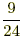or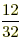etc.can be writtenor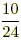or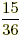or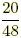etc. This shows that the least common denominator is 24.